cancel
Showing results for
Did you mean:Frequent Visitor

## Running Weekly Total Combining Two Data Sources

Hello all,

I'm looking to create a Matrix (or table) that displays Weekly Planned Targets for each week of the Quarter, which then turn into Actual numbers as the Quarter progresses. So for any week that is in the Current Week or in the future, it displays the Planned Target and if its a Week in the past, it displays the Actual achieved number.

I have this working they way I would like via a set of measures which give me the Current Week (I can therefore determine if week is in the Past/Current/Future), an Actual Qty and a Plan Qty. See the broken out tables below

1. Plan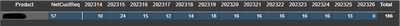2. Actual3. Actual & Plan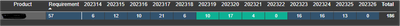However, what I am looking to do is have a running cumulative total that COMBINES my Actuals to date with my future plans to give me a total number. So in the table above (Table 3. Actual&Plan) it would total what I have actually achieved with what I'm projecting to achieve.

I have made several attempts at this with the closest output being below;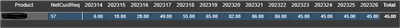As you can see this is summing up the Actuals each week, 6 in Week 1, 12 in Week 2 which is a running total of 18 etc. However this stops working when we reach the end of the actuals and doesnt then continue to sum up the Actuals to Date with the remaining Plan.

The DAX I have used for the above is;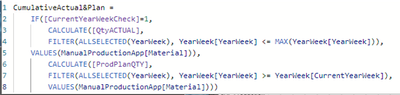Note: "CurrentWeekCheck" is bascially looking up a Fiscal Calendar and if the week is in the past, it displays 1, if its a current week or a future week, it displays 0.

I feel like I'm really close with this, but just cant quite get it over the finish line. If a fresh pair of eyes can help me do a running weekly total of BOTH Actual and Plan, I would be very grateful.

Thank you.

1 ACCEPTED SOLUTIONCommunity Support

Hi @MiloPowerBI ,

Here I create a sample to have a test.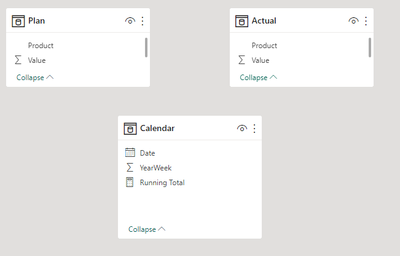Measure:

``````Running Total =
VAR _CURRENTYEARWEEK =
YEAR ( TODAY () ) * 100
+ WEEKNUM ( TODAY (), 1 )
VAR _GENERATE =
GENERATE (
CALCULATETABLE ( VALUES ( 'Calendar'[YearWeek] ), ALL ( 'Calendar' ) ),
CALCULATETABLE ( VALUES ( Actual[Product] ), ALL ( Actual[Product] ) )
)
_GENERATE,
"Value",
IF (
'Calendar'[YearWeek] < _CURRENTYEARWEEK,
CALCULATE (
SUM ( Actual[Value] ),
FILTER (
Actual,
Actual[YearWeek] = EARLIER ( [YearWeek] )
&& Actual[Product] = EARLIER ( [Product] )
)
) + 0,
CALCULATE (
SUM ( Plan[Value] ),
FILTER (
Plan,
Plan[YearWeek] = EARLIER ( [YearWeek] )
&& Plan[Product] = EARLIER ( [Product] )
)
)
)
)
VAR _MIN =
CALCULATE ( MIN ( Actual[YearWeek] ), ALLEXCEPT ( Actual, Actual[Product] ) )
VAR _MAX =
CALCULATE ( MAX ( Plan[YearWeek] ), ALLEXCEPT ( Plan, Plan[Product] ) )
RETURN
IF (
MAX ( 'Calendar'[YearWeek] ) >= _MIN
&& MAX ( 'Calendar'[YearWeek] ) <= _MAX,
SUMX (
FILTER (
[Product] = MAX ( Actual[Product] )
&& [YearWeek] <= MAX ( 'Calendar'[YearWeek] )
),
[Value]
)
)``````

Result is as below.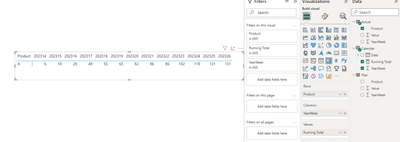Best Regards,
Rico Zhou

If this post helps, then please consider Accept it as the solution to help the other members find it more quickly.

4 REPLIES 4Community Support

Hi @MiloPowerBI ,

Here I create a sample to have a test.Measure:

``````Running Total =
VAR _CURRENTYEARWEEK =
YEAR ( TODAY () ) * 100
+ WEEKNUM ( TODAY (), 1 )
VAR _GENERATE =
GENERATE (
CALCULATETABLE ( VALUES ( 'Calendar'[YearWeek] ), ALL ( 'Calendar' ) ),
CALCULATETABLE ( VALUES ( Actual[Product] ), ALL ( Actual[Product] ) )
)
_GENERATE,
"Value",
IF (
'Calendar'[YearWeek] < _CURRENTYEARWEEK,
CALCULATE (
SUM ( Actual[Value] ),
FILTER (
Actual,
Actual[YearWeek] = EARLIER ( [YearWeek] )
&& Actual[Product] = EARLIER ( [Product] )
)
) + 0,
CALCULATE (
SUM ( Plan[Value] ),
FILTER (
Plan,
Plan[YearWeek] = EARLIER ( [YearWeek] )
&& Plan[Product] = EARLIER ( [Product] )
)
)
)
)
VAR _MIN =
CALCULATE ( MIN ( Actual[YearWeek] ), ALLEXCEPT ( Actual, Actual[Product] ) )
VAR _MAX =
CALCULATE ( MAX ( Plan[YearWeek] ), ALLEXCEPT ( Plan, Plan[Product] ) )
RETURN
IF (
MAX ( 'Calendar'[YearWeek] ) >= _MIN
&& MAX ( 'Calendar'[YearWeek] ) <= _MAX,
SUMX (
FILTER (
[Product] = MAX ( Actual[Product] )
&& [YearWeek] <= MAX ( 'Calendar'[YearWeek] )
),
[Value]
)
)``````

Result is as below.Best Regards,
Rico Zhou

If this post helps, then please consider Accept it as the solution to help the other members find it more quickly.Frequent Visitor

Hello,

Thank you - this looks very promising. Apologies for the delay in my response, I have been offline for a couple of days. I will try this today and get back to you.Super User

Please provide sample data that covers your issue or question completely, in a usable format (not as a screenshot).
https://community.fabric.microsoft.com/t5/Community-Blog/How-to-provide-sample-data-in-the-Power-BI-...
Please show the expected outcome based on the sample data you provided.Frequent Visitor

Hello lbendlin,

Apologies, I am pretty new around here and didnt realise if I could upload files with data in them. Will take this lesson forward for next time. Thank you for the helpful links.Announcements#### Exclusive opportunity for Women!

Join us for a free, hands-on Microsoft workshop led by women trainers for women where you will learn how to build a Dashboard in a Day!#### Power Platform Conference-Power BI and Fabric Sessions

Join us Oct 1 - 6 in Las Vegas for the Microsoft Power Platform Conference.Top Solution Authors
Top Kudoed Authors
Users online (3,375)